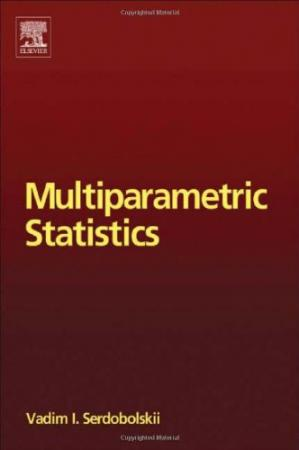## Change Language# Multiparametric statisticsAuthor(s): Vadim Ivanovich Serdobolskii Collection: Publisher: Elsevier Year: 2008 Language: English Pages: 335 pages Size: 1.49 MB Extension: PDF

[tab] [content title="Description"]This monograph presents mathematical theory of statistical models described by the essentially large number of unknown parameters, comparable with sample size but can also be much larger. In this meaning, the proposed theory can be called "essentially multiparametric". It is developed on the basis of the Kolmogorov asymptotic approach in which sample size increases along with the number of unknown parameters. This theory opens a way for solution of central problems of multivariate statistics, which up until now have not been solved. Traditional statistical methods based on the idea of an infinite sampling often break down in the solution of real problems, and, dependent on data, can be inefficient, unstable and even not applicable. In this situation, practical statisticians are forced to use various heuristic methods in the hope the will find a satisfactory solution. Mathematical theory developed in this book presents a regular technique for implementing new, more efficient versions of statistical procedures. Near exact solutions are constructed for a number of concrete multi-dimensional problems: estimation of expectation vectors, regression and discriminant analysis, and for the solution to large systems of empiric linear algebraic equations. It is remarkable that these solutions prove to be not only non-degenerating and always stable, but also near exact within a wide class of populations. In the conventional situation of small dimension and large sample size these new solutions far surpass the classical, commonly used consistent ones. It can be expected in the near future, for the most part, traditional multivariate statistical software will be replaced by the always reliable and more efficient versions of statistical procedures implemented by the technology described in this book. This monograph will be of interest to a variety of specialists working with the theory of statistical methods and its applications. Mathematicians would find new classes of urgent problems to be solved in their own regions. Specialists in applied statistics creating statistical packages will be interested in more efficient methods proposed in the book. Advantages of these methods are obvious: the user is liberated from the permanent uncertainty of possible instability and inefficiency and gets algorithms with unimprovable accuracy and guaranteed for a wide class of distributions. A large community of specialists applying statistical methods to real data will find a number of always stable highly accurate versions of algorithms that will help them to better solve their scientific or economic problems. Students and postgraduates will be interested in this book as it will help them get at the foremost frontier of modern statistical science. - Presents original mathematical investigations and open a new branch of mathematical statistics - Illustrates a technique for developing always stable and efficient versions of multivariate statistical analysis for large-dimensional problems - Describes the most popular methods some near exact solutions; including algorithms of non-degenerating large-dimensional discriminant and regression analysis [/content] [content title="Content"] [/content] [content title="About the author"]Vadim Ivanovich Utkin (Russian: Вадим Иванович Уткин; born 1937) is a Russian-American control theorist, electrical engineer and a current professor of Electrical Engineering and Mechanical Engineering at the Ohio State University. He is best known for being one of the originators of Sliding Mode Control and Variable Structure Systems, [/content] [/tab]